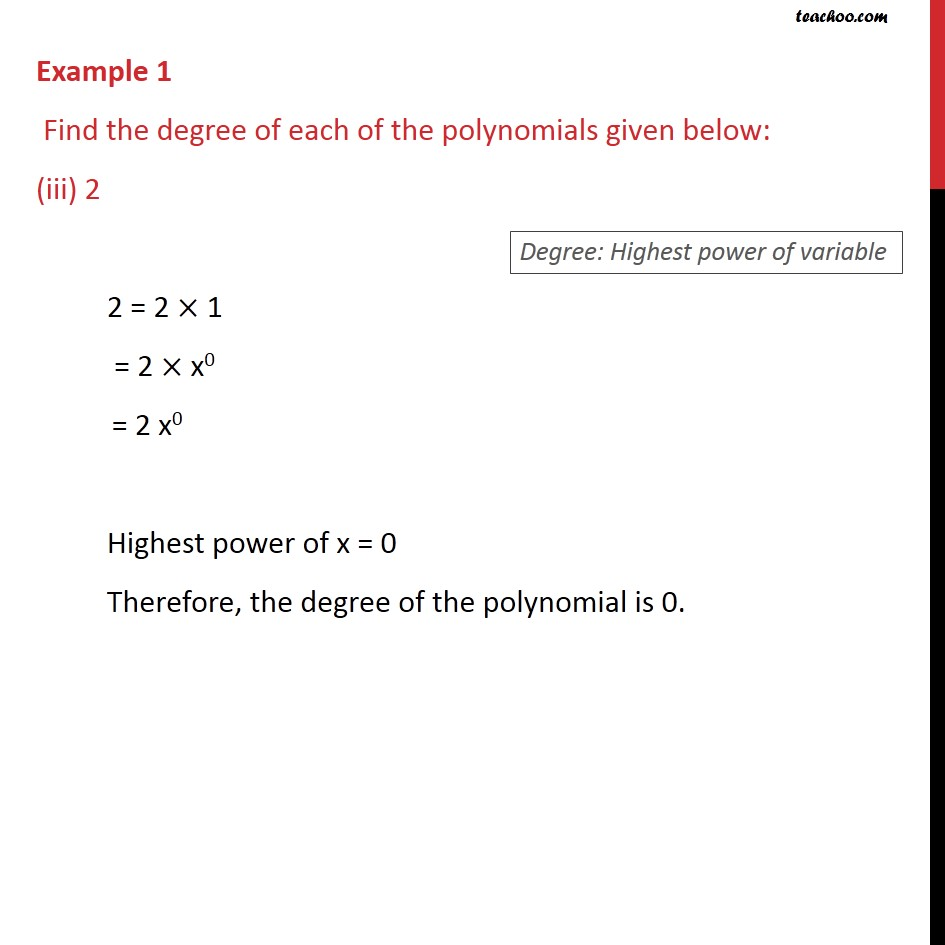Examples

Chapter 2 Class 9 Polynomials
Serial order wiseLearn in your speed, with individual attention - Teachoo Maths 1-on-1 Class

### Transcript

Example 1 Find the degree of each of the polynomials given below: (iii) 2 2 = 2 1 = 2 x0 = 2 x0 Highest power of x = 0 Therefore, the degree of the polynomial is 0.